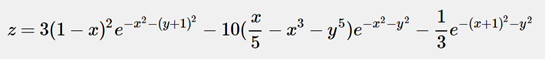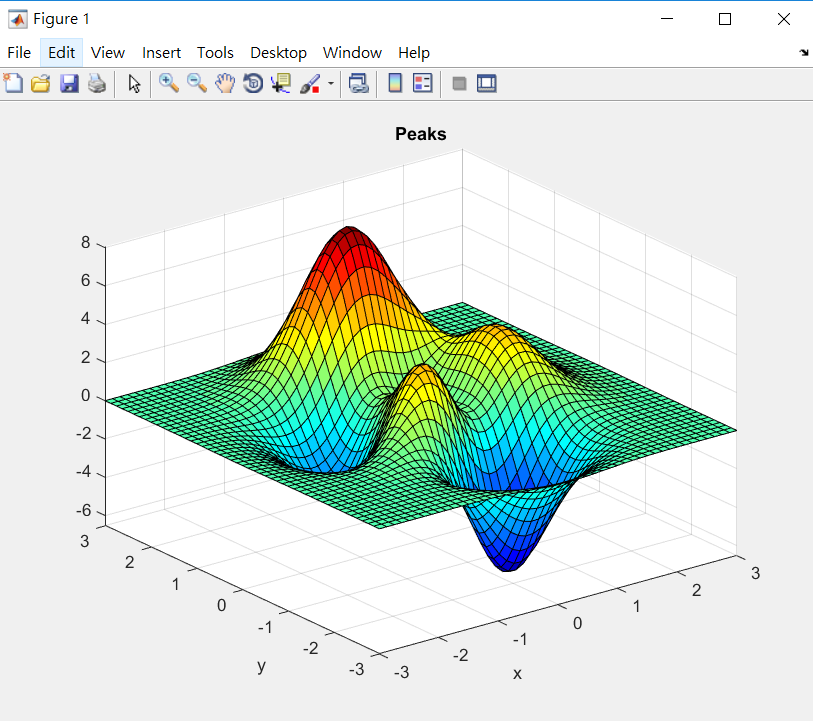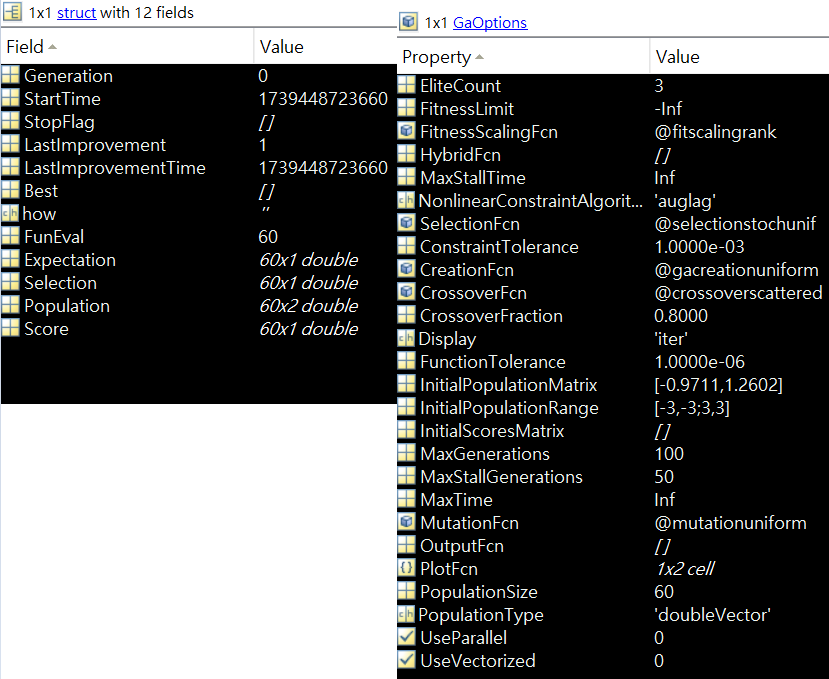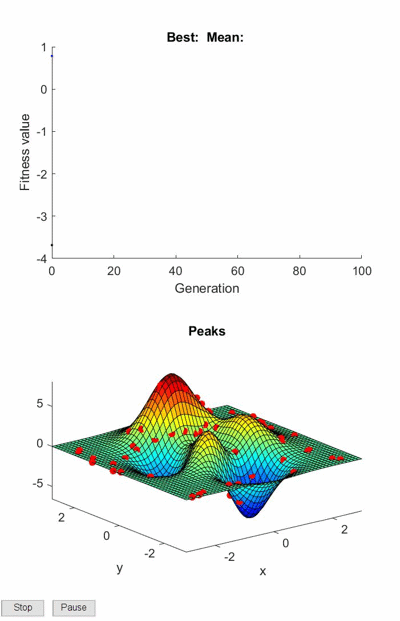• 6663
• 0
• GA
• 2019-11-18

## 目次

1. 【MATLAB】應用基因演算法(一)：Peaks 函數求極值
2. 【MATLAB】應用基因演算法(二)：找尋正確字串
3. 【MATLAB】應用基因演算法(三)：高效率的送貨員

## 1. GA 調用方法

MATLAB                                     Version 9.3         (R2017b)
Optimization Toolbox                  Version 8.0         (R2017b)
Global Optimization Toolbox       Version 3.4.3      (R2017b)

x = ga(fitnessfcn,nvars)
x = ga(fitnessfcn,nvars,A,b)
x = ga(fitnessfcn,nvars,A,b,Aeq,beq)
x = ga(fitnessfcn,nvars,A,b,Aeq,beq,LB,UB)
x = ga(fitnessfcn,nvars,A,b,Aeq,beq,LB,UB,nonlcon)
x = ga(fitnessfcn,nvars,A,b,Aeq,beq,LB,UB,nonlcon,options)
x = ga(fitnessfcn,nvars,A,b,[],[],LB,UB,nonlcon,IntCon)
x = ga(fitnessfcn,nvars,A,b,[],[],LB,UB,nonlcon,IntCon,options)

[x,fval] = ga(fitnessfcn,nvars,...)
[x,fval,exitflag] = ga(fitnessfcn,nvars,...)
[x,fval,exitflag,output] = ga(fitnessfcn,nvars,...)
[x,fval,exitflag,output,population] = ga(fitnessfcn,nvars,...)
[x,fval,exitflag,output,population,scores] = ga(fitnessfcn,nvars,...)


[x, fval] = ga(fitnessfun, nvars, A, B, C, D, LB, UB, [ ], IntCon, options)

[最佳解, 適應值] = ga(適應函數，變數數量，A，B，C，D，下界，上界，非線性約束式，整數欄位，參數)

## 2. Peaks 函數求最小值options = optimoptions( ...
'ga', ...                                    % 最佳化算法
'PopulationSize', 60, ...                    % 染色體數量
'MaxGenerations', 100, ...                   % 最大繁衍代數
'PlotFcn', {@gaplotbestf, @my_plot}, ...     % 繪圖函數
'CrossoverFraction', 0.8, ...                % 交配率
'Display', 'iter');                          % 結果展示方式

% 突變率設定方式
% options = optimoptions('MutationFcn', {@mutationuniform, 0.1})

※ 2019.07.24 更新 ※

## 3. 適應函數 & 自定義繪圖函數

% 自定義適應函數
function Z = FitnessFcn(x)

X = x(1);
Y = x(2);

% 以下為 Peaks 函數
Z =  3*(1-X).^2.*exp(-(X.^2) - (Y+1).^2) ...
- 10*(X/5 - X.^3 - Y.^5).*exp(-X.^2-Y.^2) ...
- 1/3*exp(-(X+1).^2 - Y.^2);
end

MATLAB 提供很多繪圖的函數供使用者挑選，比較常見的有：

1. @gaplotbestf               ：最佳分數及平均分數
2. @gaplotscores            ：每代個體的分數
3. @gaplotdistance         ：每代個體間的平均距離
4. @gaplotscorediversity：繪製每一代分數的直方圖

### function state = plotfun(options,state,flag)

options：最新的參數設定。
state     ：最新子代的資訊。
flag       ：目前算法的狀態，例如：'init'、'iter'...等。% 自定義繪圖函數，畫出每次迭代的結果。
function state = my_plot(options, state, flag)

% 繪製所有點位的適應值
X = state.Population(:, 1);
Y = state.Population(:, 2);
Z =  3*(1-X).^2.*exp(-(X.^2) - (Y+1).^2) ...
- 10*(X/5 - X.^3 - Y.^5).*exp(-X.^2-Y.^2) ...
- 1/3*exp(-(X+1).^2 - Y.^2);

% 叫出 peaks 圖形
peaks;

% 把所有適應值，繪製到同一張圖面上
hold on
plot3(X, Y, Z, 'r.', 'MarkerSize', 10, 'Parent', gca)
hold off
end

% 第一項輸入值為適應函數
% 第二項為最佳化的參數個數，在本範例中有X與Y，故輸入2
% 第三～六項為限制式
% 第七、八項為下界與上界
% 第九項為非線性約束式
% 第十項為整數欄位，在本範例中，並不限制一定為整數。
% 第十一項為剛才設定的 GA 參數。
[x, fval] = ga(@FitnessFcn, 2, [], [], [], [], [-3, -3], [3, 3], [], [], options);【MATLAB】應用基因演算法(二)：找尋正確字串

## 附錄

%% Peaks 函數求極值
% 功能：如標題
%
% 2018.03.02, Shayne.

options = optimoptions( ...
'ga', ...                                    % 最佳化算法
'PopulationSize', 60, ...                    % 染色體數量
'MaxGenerations', 100, ...                   % 最大繁衍代數
'PlotFcn', {@gaplotbestf, @my_plot}, ...     % 繪圖函數
'CrossoverFraction', 0.8, ...                % 交配率
'Display', 'iter');                          % 結果展示方式

[x, fval] = ga(@FitnessFcn, 2, [], [], [], [], [-3, -3], [3, 3], [], [], options);

% 自定義適應函數
function Z = FitnessFcn(x)

X = x(1);
Y = x(2);

% 以下為 Peaks 函數
Z =  3*(1-X).^2.*exp(-(X.^2) - (Y+1).^2) ...
- 10*(X/5 - X.^3 - Y.^5).*exp(-X.^2-Y.^2) ...
- 1/3*exp(-(X+1).^2 - Y.^2);
end

% 自定義繪圖函數，畫出每次迭代的結果。
function state = my_plot(options, state, flag)

% 繪製所有點位的適應值
X = state.Population(:, 1);
Y = state.Population(:, 2);
Z =  3*(1-X).^2.*exp(-(X.^2) - (Y+1).^2) ...
- 10*(X/5 - X.^3 - Y.^5).*exp(-X.^2-Y.^2) ...
- 1/3*exp(-(X+1).^2 - Y.^2);

% 叫出 peaks 圖形
peaks;

% 把所有適應值，繪製到同一張圖面上
hold on
plot3(X, Y, Z, 'r.', 'MarkerSize', 10, 'Parent', gca)
hold off
end